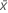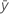Methods and formulas for Correlation

Select the method or formula of your choice.

P-value

P-values are often used in hypothesis tests to determine whether you reject or fail to reject the null hypothesis.

For Pearson's correlation coefficient:

H0: ρ = 0 versus H1: ρ ≠ 0 where ρ is the correlation coefficient between a pair of variables.

A small p-value is an indication that the null hypothesis is false. You can conclude that the correlation coefficient is different from zero and that a linear relationship exists. It is common to reject the null hypothesis if the p-value is smaller than 0.05.

Formula

The p-value for Pearson's correlation coefficient uses the t-distribution.

The p-value is 2 × P(T > t) where T follows a t distribution with n – 2 degrees of freedom.

Notation

TermDescription
rcorrelation coefficient
nnumber of observations

Pearson's correlation coefficient

Formula

Measures the degree of linear relationship between two variables. The correlation coefficient assumes a value between −1 and +1. If one variable tends to increase as the other decreases, the correlation coefficient is negative. Conversely, if the two variables tend to increase together the correlation coefficient is positive.

For the variables x and y:

Notation

TermDescriptionsample mean for the first variable
sx standard deviation for the first variablesample mean for the second variable
sy standard deviation for the second variable
n column length

Spearman's correlation coefficient

To calculate Spearman's correlation coefficient and p-value, perform a Pearson correlation on the ranks of the data. The ranks of tied responses are the average of the ranks of the ties. The table that follows shows the ranks for two samples of data.

C1 C2 C3 C4
A Rank A B Rank B
45 4 23 1
78 6 25 3
24 3 25 3
51 5 25 3
13 1.5 34 6
13 1.5 30 5

Spearman's correlation coefficient between A and B is −0.678 and the p-value is 0.139. These values are identical to the coefficient and p-value from a Pearson correlation on the values in Rank A and Rank B.

Minitab omits rows that contain missing data for one or both variables from the calculations. Both columns must have the same number of rows.

By using this site you agree to the use of cookies for analytics and personalized content.  Read our policy